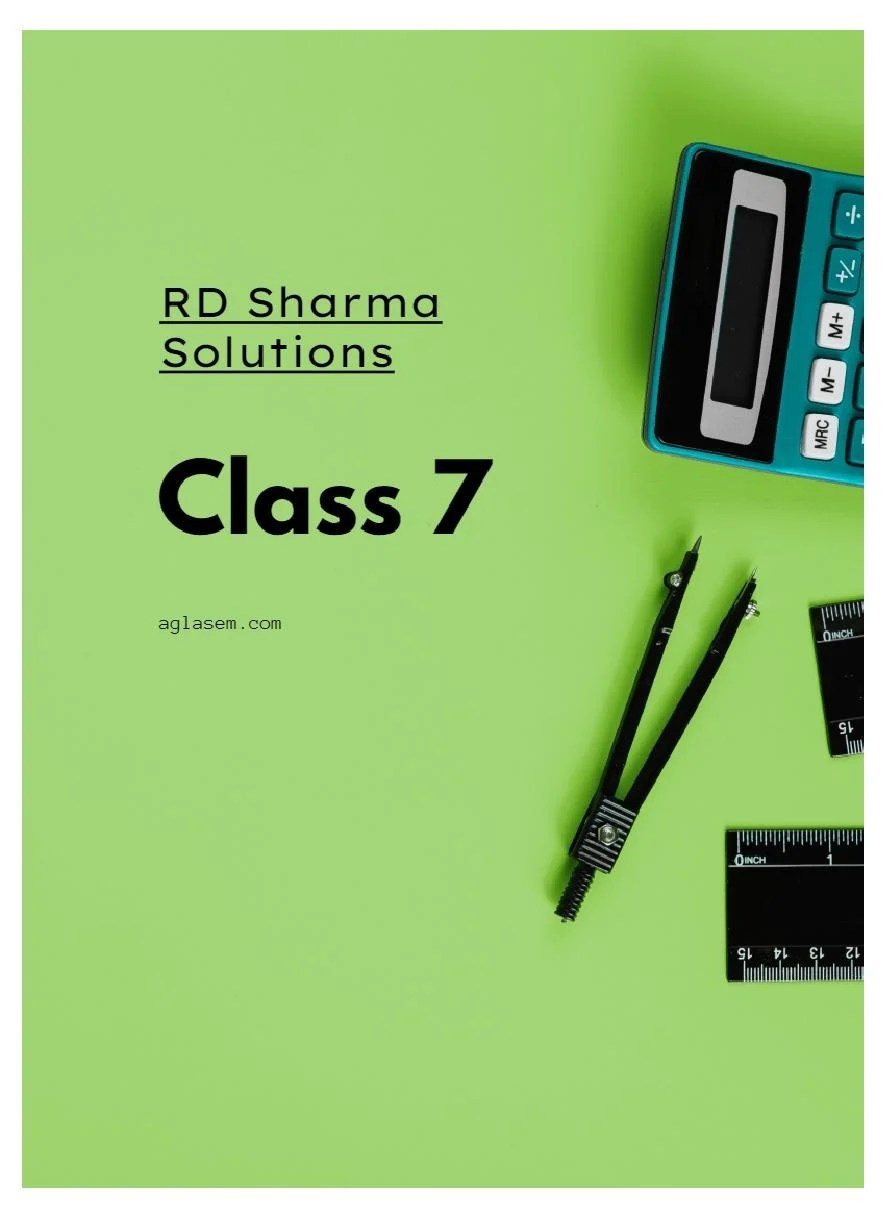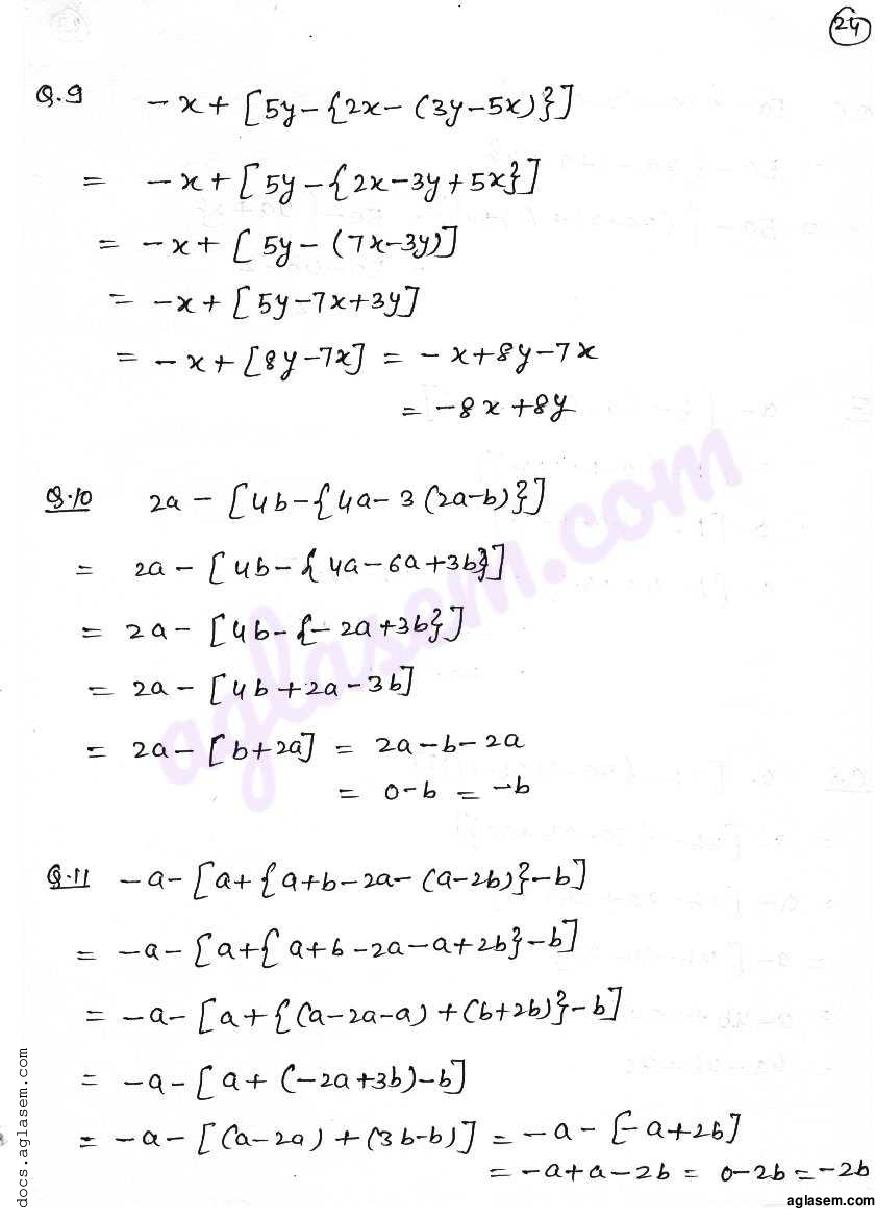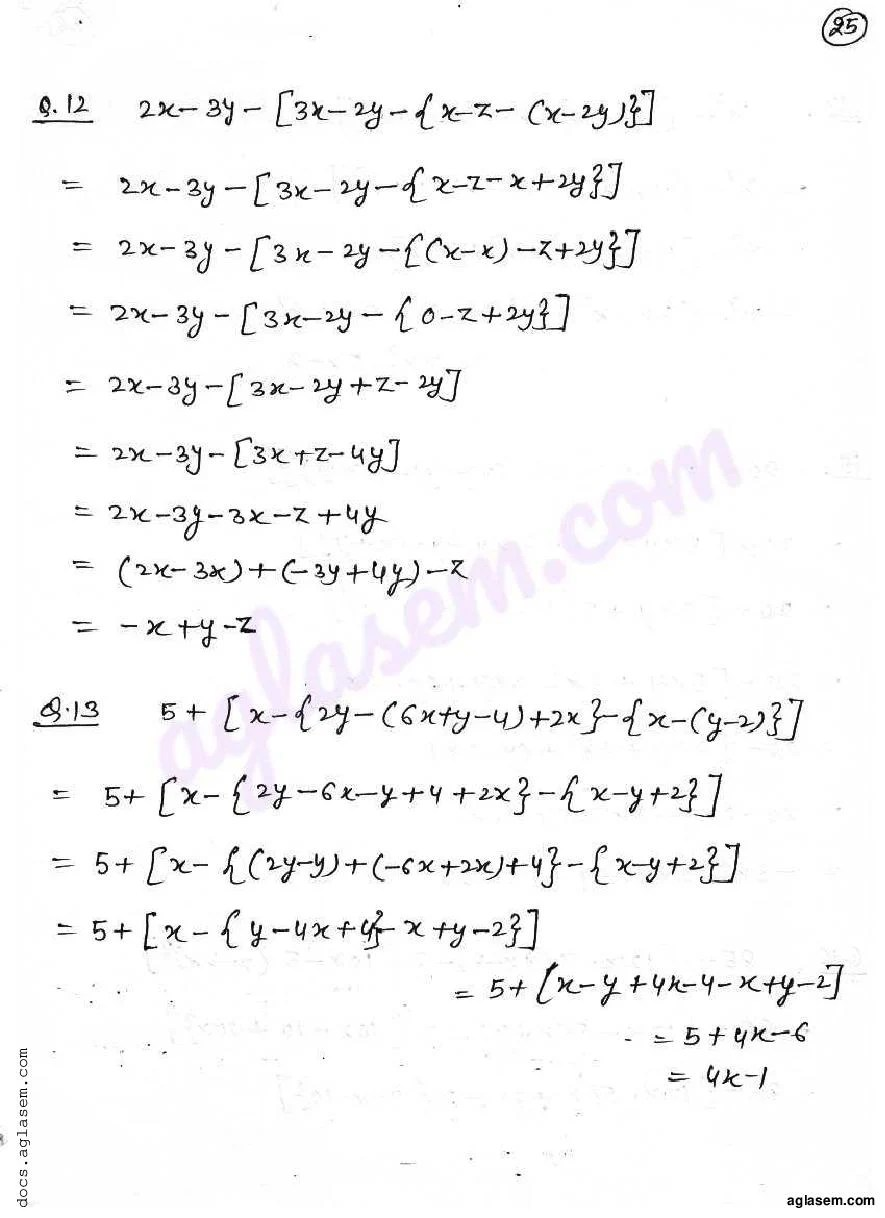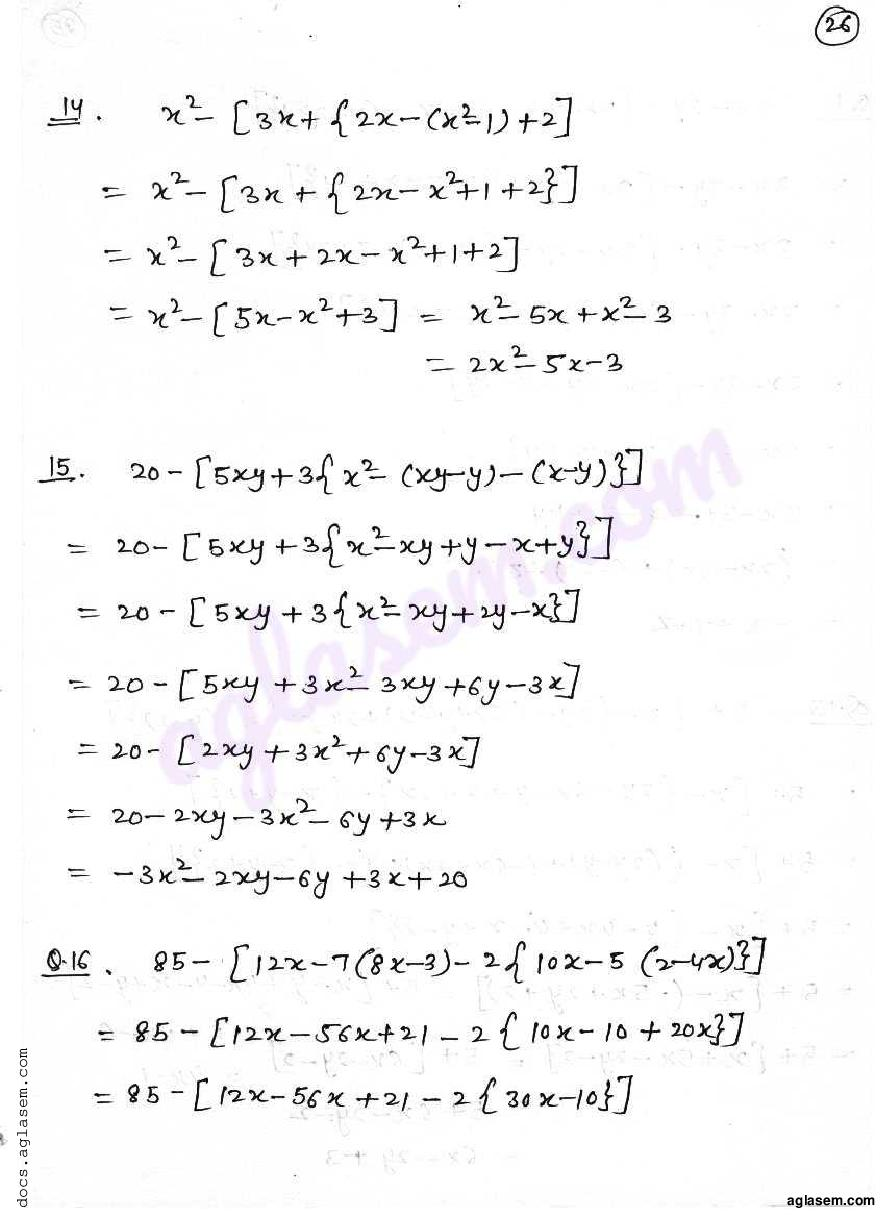# RD Sharma Solutions Class 7 Chapter 7 Algebraic Expressions Exercise 7.4

Here you can get free RD Sharma Solutions for Class 7 Maths Chapter 7 Algebraic Expressions Exercise 7.4. All RD Sharma Book Solutions are given here exercise wise for the chapter Algebraic Expressions. RD Sharma Solutions are helpful in the preparation of several school level, graduate and undergraduate level competitive exams. Practicing questions from RD Sharma Mathematics Solutions for Class 7 Chapter 7 Algebraic Expressions is proven to enhance your math skills.

 Class: Class 7th Chapter: Chapter 7 Exercise: Exercise 7.4 Name: Algebraic Expressions

## RD Sharma Solutions Class 7 Chapter 7 Algebraic Expressions Exercise 7.4

RD Sharma Class 7 Solutions Chapter 7 for Algebraic Expressions Exercise 7.4 are given below.

RD Sharma Solutions Class 7 Chapter 7 Algebraic Expressions Exercise 7.4